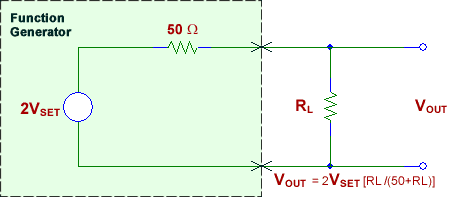ECE 392 - Electrical Engineering Laboratory II

# Measurement Tips

## Tip 1

Most function generators are designed with a 50-ohm output Impedance - there is a50-ohm resistor inside the function generator that is in series with the output. This design helps to minimize signal reflections when the output is connected to a coax cable that has a characteristic impedance of 50-ohms and is terminated with a 50-ohm load. The 50-ohm output impedance and 50-ohm load form a 2-to-1 voltage divider. Therefore, the actual internal function generator voltage that is produced is twice your set value, VSET, to produce an output voltage on the 50-ohm load equal to your setting. Note that if the load resistance is infinite (an open circuit), the resulting output voltage will be twice your setting. And If the load resistance, RL, is any value other than 50 ohms, the actual output voltage, VOUT, will be:

VOUT =2VSET [RL /(50+RL)] See figureEffect of function generator 50 Ω output impedance on output voltage.

If you set a voltage VSET with RL = 50 Ω : VOUT = 2VSET [50 /(50+50)] = VSET

If you set a voltage VSET with RL = ∞ Ω : VOUT = 2VSET [∞ /(50+∞)] = 2VSET

## Tip 2

Agilent 33210A, 33220A, and 33250A function/arbitrary wave-form generators allow you to enter a value for the expected load resistance, RL, in the range of 1ohm to 10k ohms, or infinite. The default value is 50 ohms. If you change the value for this parameter, the function generator will automatically adjust the internal voltage it produces to take into consideration the voltage divider formed by the internal 50-ohm resistor and your load resistance such that VOUT is equal to your voltage setting. This adjustment applies to both the DC offset and the AC portion of the function generator output signal.

From Agilent Technologies
Measurement Tips
Volume 6, Number 2